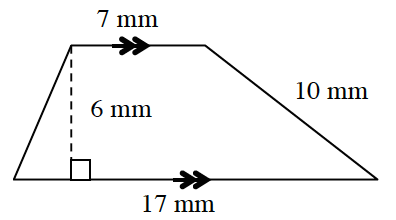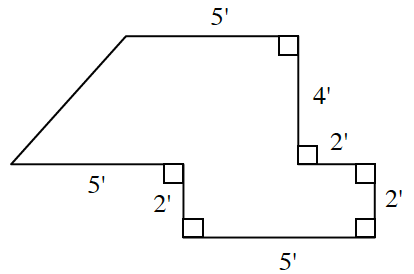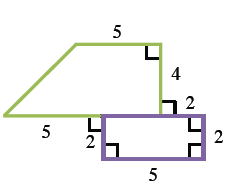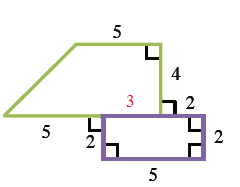### Home > INT1 > Chapter Ch10 > Lesson 10.1.2 > Problem10-38

10-38.

Find the area and perimeter of each polygon below. Show all work. Homework Help ✎

1.1.Divide the figure into two separate shapes.
Afterwards, find the area for each shape and then add them together.

The piece shared between the trapezoid and the rectangle is 5 - 2 = 3 because the red rectangle has side 5. This makes the base of the trapezoid 8.

Area of Shape = Area of Trapezoid + Area of Rectangle

$\frac{1}{2}\left(\textit{b}_1+\textit{b}_2\right)+\textit{bh}$

$\frac{1}{2}([5+3]+5)4+2\cdot 5$

Total area = 36 units²